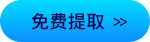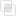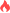python统计不同字符的个数

Python 字符2021-04-28 09:45:031225浏览 · 0收藏 · 0评论1、统计过程

2、实例

```n=input("请输入一行字符：")
#输入一行字符
a=b=c=d=0
#a为字母的个数，b为数字的个数，c为空格的个数,d为其他字符的个数
for i in n:
#for循环遍历字符串n
#ord()内置函数，返回对应的ASCII数值
if ord('a')<=ord(i)<=ord('z') or ord('A')<=ord(i)<=ord('Z'):
#返回的数值在字符a,z数值之间，则i为字符a,z之间的字母，其他同理
a=a+1
elif ord('0')<=ord(i)<=ord('9'):
b=b+1
elif ord(i)==ord(' '):
c=c+1
else:
d=d+1
print("这一行字符串中字母的数量是：{},数字的数量是:{},空格的数量是:{},其他字符的数量是:{}。".format(a,b,c,d))```django中如何显示字符串？python 字符串最常用的13个处理操作python【图片转字符画】，图片灰度值处理leetcode题目：880.索引处的解码字符串知道Python中的字符串是什么吗？python文本字符处理的简单方法Python3：内置数据结构--字符串、列表、元组、集合、字典python查询字符串参数python查找两文本不同字符及其相对重复率等及其pyqt5界面php包含字符python字符串如何取值python字符串格式化的方法整理python字符串如何简单运算php数组转json字符串python使用f格式化字符串python中str()函数转换字符串java反射调用指定的属性258

java获取class实例的方式636

java中反射提供哪些功能867